# Measuring Length Worksheets for Grade 3

Download Measuring Length Worksheets for Grade 3Q.1) Convert:
5 km = __ m

Q.2) Convert:
5 km 438 m = __ m

Q.3) Convert:
4000 m = __ km

Q.4) Convert:
1865 km = __ km __ m
a) 1, 865
b) 186, 5

Q.5) Convert:
7 m = __ cm

Q.6) Convert:
5 m 624 cm = __ cm

Q.7) Convert:
300 cm = __ m

Q.8) Convert:
714 cm = __ m __ cm
a) 7 , 14
b) 71 , 4

Q.9) Add 63 km 745 m and 25 km 452 m ?
a) 85 km 68 m
b) 89 km 197 m
c) 86 km 137 m

Q.10) Add 23 m 35 cm and 42 m 76 cm ?
a) 64 m 17 cm
b) 69 m 13 cm
c) 66 m 11 cm

Q.11) Subtract:
79 m 87 cm – 56 m 76 cm ?
a) 19 m 18 cm
b) 21 m 6 cm
c) 23 m 11 cm

Q.12) Subtract:
65 m 48 cm – 26 m 34 cm ?
a) 39 m 14 cm
b) 36 m 56 cm
c) 41 m 23 cm

Q.13) Add 32 km 323 m and 12 km 411 m ?
a) 44 km 734 m
b) 32 km 813 m
c) 36 km 515 m

Q.14) Add 35 km 625 m and 24 km 545 m ?
a) 63 km 224 m
b) 65 km 142 m
c) 60 km 170 m

Q.15) Subtract:
97 km 769 m – 51 km 428 m ?
a) 48 km 302 m
b) 49 km 399 m
c) 46 km 341 m

### Measuring Length Worksheets for Grade 3 Explanations

Q.1) Explanation – Measuring Length Worksheets for Grade 3

As we know that,
1 km = 1000 m
To find 5 km in m. We have to multiply both sides by 5
( 5 x 1 ) km = ( 5 x 1000 ) m
5 km = 5000 m

Q.2) Explanation – Measuring Length Worksheets for Grade 3

5 km 438 m can also be written as 5 km + 438 m
To change 5 km + 438 m into m
we have to convert 5 km in m
As we know that,
1 km = 1000 m
5 km = ( 5 x 1000 ) m
5 km = 5000 m
Therefore,
5 km + 438 m = 5000 m + 438 m
= 5438 m

Q.3) Explanation – Measuring Length Worksheets for Grade 3

As we know that,
1 km = 1000 m
or 1000 m = 1km
then,
1 m = 1/1000 km

To find 4000 m in km. We have to multiply both sides by 4000
( 4000 x 1 ) m = ( 4000 x 1/1000 )km

4000 m = 4 km

Q.4) Explanation – Measuring Length Worksheets for Grade 3

As we know that,
1 km = 1000 m
or 1000 m = 1 km
then,
1 m = 1/1000 km

To find 1865 km in km and m. We have to multiply both sides by 1865
( 1865 x 1 ) m = ( 1865 x 1/1000 )km

1865 m = 1.865 km
Digits before decimal point is written with kilometre ( km ) and after decimal point with metre ( m )
Hence, 1865 km = 1 km 865 m

Correct Answer – a) 1, 865

Q.5) Explanation – Measuring Length Worksheets for Grade 3

As we know that,
1 m = 100 cm
To find 7 m in cm. We have to multiply both sides by 7
( 7 x 1 ) m = ( 7 x 100 ) cm
7 m = 700 cm

Q.6) Explanation –

5 m 624 cm can also be written as 5 m + 624 cm
To change 5 m + 624 cm into cm
we have to convert 5 m in cm
As we know that,
1 m = 100 cm
5 m = ( 5 x 100 ) cm
5 m = 500 cm
Therefore,
5 m + 624 cm = 500 cm + 624 cm
= 1124 cm

Q.7) Explanation – Measuring Length Worksheets for Grade 3

As we know that,
1 m = 100 cm
or 100 cm = 1m
then,
1 cm = 1/100 m

To find 300 cm in m. We have to multiply both sides by 300
( 300 x 1 ) cm = ( 300 x 1/100 )m
300 cm = 3 m

Q.8) Explanation –

As we know that,
1 m = 100 cm
or 100 cm = 1 m
then,
1 cm = 1/100 m

To find 714 cm in m and cm. We have to multiply both sides by 714
( 714 x 1 ) cm = ( 714 x 1/100 )m
714 cm = 7.14 m
Digits before decimal point is written with metre ( m ) and after decimal point with centimetre ( cm )
Hence, 714 cm = 7 m 14 cm

Correct Answer – a) 7 , 14

Q.9) Explanation – Measuring Length Worksheets for Grade 3

Write Kilometres (km) and Metres (m) aligned in columns properly.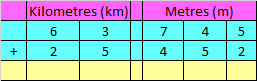Add Kilometres (km) and Metres (m) as we add numbers in Addition.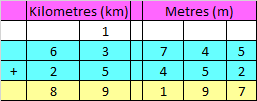Hence, the sum is 89 km 197 m

Correct Answer – b) 89 km 197 m

Q.10) Explanation –

Step I: Write m and cm aligned in columns properly.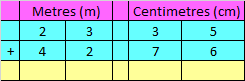Step II: Add Metres (m) and Centimetres (cm) as we add numbers in Addition.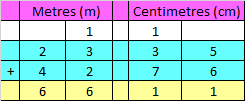Hence, the sum is 66 m 11 cm

Correct Answer – c) 66 m 11 cm

Q.11) Explanation – Measuring Length Worksheets for Grade 3

Write Metres (m) and Centimetres (cm) aligned in columns properly.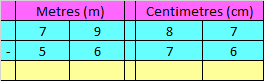Subtract Metres (m) and Centimetres (cm) as we subtract numbers in Subtraction.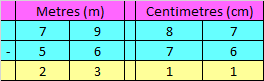Hence, the difference is 23 m 11 cm

Correct Answer – c) 23 m 11 cm

Q.12) Explanation –

Step I:Write Metres (m) and Centimetres (cm) aligned in columns properly.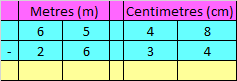Step II:Subtract Metres (m) and Centimetres (cm) as we subtract numbers in Subtraction.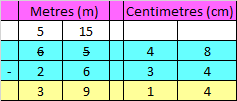Hence, the difference is 39 m 14 cm

Correct Answer – a) 39 m 14 cm

Q.13) Explanation – Measuring Length Worksheets for Grade 3

Write Kilometres (km) and Metres (m) aligned in columns properly.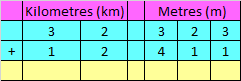Add Kilometres (km) and Metres (m) as we add numbers in Addition.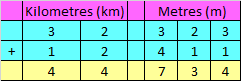Hence, the sum is 44 km 734 m

Correct Answer – a) 44 km 734 m

Q.14) Explanation –

Write Kilometres (km) and Metres (m) aligned in columns properly.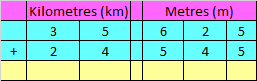Add Kilometres (km) and Metres (m) as we add numbers in Addition.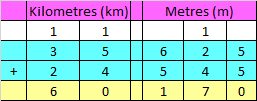Hence, the sum is 60 km 170 m

Correct Answer – c) 60 km 170 m

Q.15) Explanation – Measuring Length Worksheets for Grade 3

Write Kilometres (km) and Metres (m) aligned in columns properly.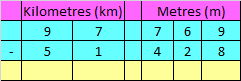Subtract Kilometres (km) and Metres (m) as we subtract numbers in Subtraction​.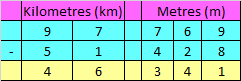Hence, the difference is 46 km 341 m

Correct Answer – c) 46 km 341 m